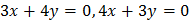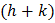# The sides of a trianglelie on the linesand. letbe the centre of the circle inscribed in. The value ofequals

## Question ID - 153590 :- The sides of a trianglelie on the linesand. letbe the centre of the circle inscribed in. The value ofequals

3537

(0)

Equation of angle bisector of angleEquation of internal bisector isSinceandlie on the lineNext Question :
 A man starts from the pointand reaches pointtouching-axis atsuch thatis minimum, then